# 机器学习分类算法常用评价指标

1.  准确率，召回率，精确率，F1-score，Fβ，ROC曲线，AUC值

2. 宏平均（Macro-averaging）和微平均（Micro-averaging）

3. Python3  sklearn实现分类评价指标

## 1.  准确率，召回率，精确率，F1-score，Fβ，ROC曲线，AUC值

为了评价模型以及在不同研究者之间进行性能比较，需要统一的评价标准。根据数据挖掘理论的一般方法，评价模型预测能力最广泛使用的是二维混淆矩阵（Confusion matrix）（如下表所示）。

二维混淆矩阵

 真实类别 预测结果 类别1（正例） 类别2（反例） 类别1（正例） 真正例(True Positive) TP 假反例(False Negatibe) FN 类别2（反例） 假正例(False Positive)FP 真反例(True Negatibe) TN

（1）准确率（Accuracy）表示正确分类的测试实例的个数占测试实例总数的比例，计算公式为：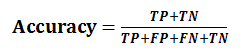（2）召回率（Recall），也叫查全率，表示正确分类的正例个数占实际正例个数的比例，计算公式为：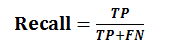（3）精确率（Precision），也叫查准率，表示正确分类的正例个数占分类为正例的实例个数的比例，计算公式为：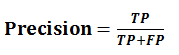（4）F1-score是基于召回率（Recall）与精确率（Precision）的调和平均，即将召回率和精确率综合起来评价，计算公式为: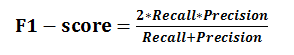（5） Fβ加权调和平均

Fβ是F1度量的一般形式，能让我们表达出对查准率、查全率的不同偏好,计算公式如下：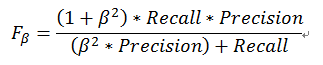其中，β>0度量了查全率对查准率的相对重要性。β=1时退化为标准的F1；β>1时查全率有更大影响；β<1时查准率有更大影响。

（6）度量分类中的非均衡性的工具ROC曲线（ROC Curve）

TPR（True Positive Rate）表示在所有实际为阳性的样本中，被正确地判断为阳性的比率，即：TPR=TP/(TP+FN)；    FPR（ False Positive Rate）表示在所有实际为阴性的样本中，被错误地判断为阳性的比率，即：FPR=FP/(FP+TN)

ROC曲线是以FPR作为X轴TPR作为Y轴。FPR越大表明预测正类中实际负类越多，TPR越大，预测正类中实际正类越多。ROC曲线如下图所示：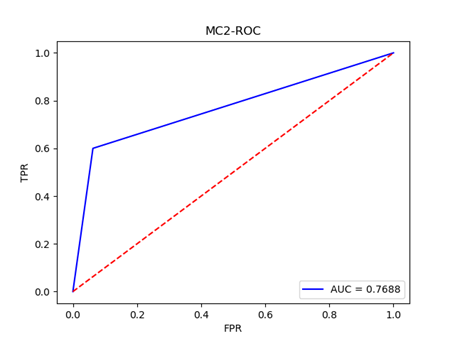（7）AUC值（Area Unser the Curve）是ROC曲线下的面积，AUC值给出的是分类器的平均性能值。使用AUC值可以评估二分类问题分类效果的优劣，计算公式如下：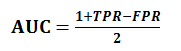一个完美的分类器的AUC为1.0，而随机猜测的AUC为0.5，显然AUC值在0和1之间，并且数值越高，代表模型的性能越好。

## 2. 宏平均（Macro-averaging）和微平均（Micro-averaging）

在n个二分类混淆矩阵上综合考虑查准率和查全率时使用。

（1）宏平均（macro-ave）

先在各混淆矩阵上分别计算出查准率，查全率和F1，然后再计算平均值，这样就得到“宏查准率”（macro-P）、“宏查全率”（macro-R）、“宏F1”（macro-F1），计算公式分别如下：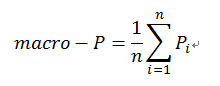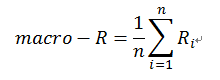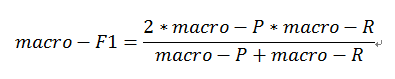（2）微平均（micro-ave）

先将各混淆矩阵的对应元素进行平均，得到TP、FP、TN、FN的平均值，再基于这些平均值计算出“微查准率”（micro-P）、“微查全率”（micro-R）、“微F1”（micro-F1）,计算公式分别如下：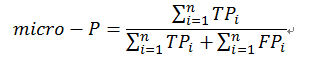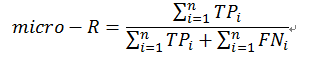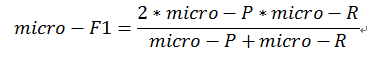## 3. Python3  sklearn实现分类评价指标

（1）KC4数据准备：KC4数据下载

（2）使用随机森林实现分类并输出评价指标

# -*- coding: utf-8 -*-

import pandas as pd
from sklearn.ensemble import RandomForestClassifier
from sklearn import metrics
import matplotlib.pyplot as plt

"""

Parameters:
filename:数据文件
Returns:
list_datasets：数据集特征列表
category_labels:数据标签列表
"""
def data_handle(filename):
list_datasets = []
category_labels = []
list_data = []
for j in range(len(read_data.iloc[i, :]) - 1):
row_data = read_data.iloc[i, j]  # 读取每个样本的每个数据
list_data.append(row_data)  #将每个数据存入列表
list_datasets.append(list_data)  #将每个样本的数据存入列表

if row_data_label == 'N':
category_labels.append(0)  # 将二分类标签转化为0和1,0代表软件正常，1代表软件缺陷
else:
category_labels.append(1)
return list_datasets, category_labels

"""

Parameters:
labels:测试标签列表
predict_prob:预测标签列表
"""
def plot_roc(labels, predict_prob):
false_positive_rate, true_positive_rate, thresholds = metrics.roc_curve(labels, predict_prob)
roc_auc = metrics.auc(false_positive_rate, true_positive_rate)  #计算AUC值
print('AUC=' + str(roc_auc))
plt.title('PC5-ROC')
plt.plot(false_positive_rate, true_positive_rate, 'b', label='AUC = %0.4f' % roc_auc)
plt.legend(loc='lower right')
plt.plot([0, 1], [0, 1], 'r--')
plt.ylabel('TPR')
plt.xlabel('FPR')
# plt.savefig('figures/PC5.png') #将ROC图片进行保存
plt.show()

if __name__ == '__main__':
datasets, labels = data_handle('MDP/KC4.csv')  # 对数据集进行处理
# 训练集和测试集划分
X_train = datasets[:115]
y_train = labels[:115]
X_test = datasets[90:]
y_test = labels[90:]
# 随机森林分类器
clf = RandomForestClassifier()
clf = RandomForestClassifier(n_estimators=200, random_state=0)
clf.fit(X_train, y_train)  # 使用训练集对分类器训练
y_predict = clf.predict(X_test)  # 使用分类器对测试集进行预测

print('准确率:', metrics.accuracy_score(y_test, y_predict)) #预测准确率输出
print('宏平均精确率:',metrics.precision_score(y_test,y_predict,average='macro')) #预测宏平均精确率输出
print('微平均精确率:', metrics.precision_score(y_test, y_predict, average='micro')) #预测微平均精确率输出
print('宏平均召回率:',metrics.recall_score(y_test,y_predict,average='macro'))#预测宏平均召回率输出
print('平均F1-score:',metrics.f1_score(y_test,y_predict,average='weighted'))#预测平均f1-score输出
print('混淆矩阵输出:',metrics.confusion_matrix(y_test,y_predict))#混淆矩阵输出

print('分类报告:', metrics.classification_report(y_test, y_predict))#分类报告输出
plot_roc(y_test, y_predict)  #绘制ROC曲线并求出AUC值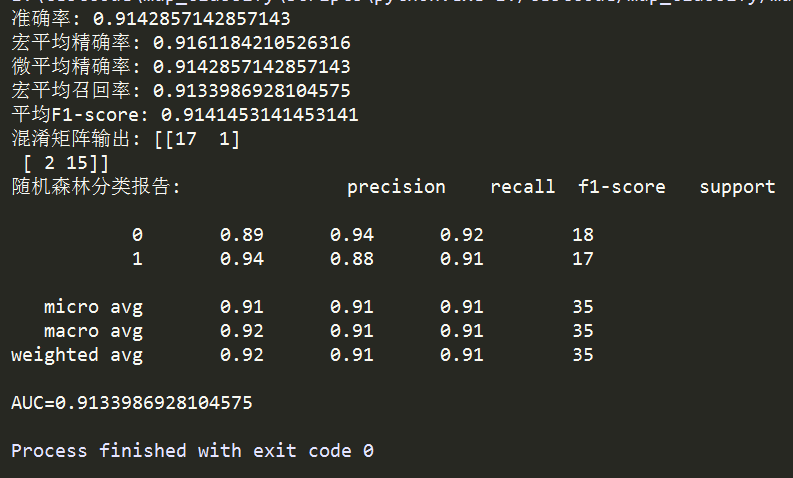### 交流学习资料共享欢迎入QQ群：955817470

09-185万+07-283万+
02-282427
11-26224
08-16123
04-061万+
04-114939
05-15
03-043940
12-25982
09-12850
08-135058
08-301368
08-151万+
10-08318
03-222389
12-18163
06-131220
04-213万+